# ABS Function – Absolute Value in Excel, VBA, Google Sheets

This tutorial demonstrates how to use the Excel ABS Function in Excel to calculate the absolute value.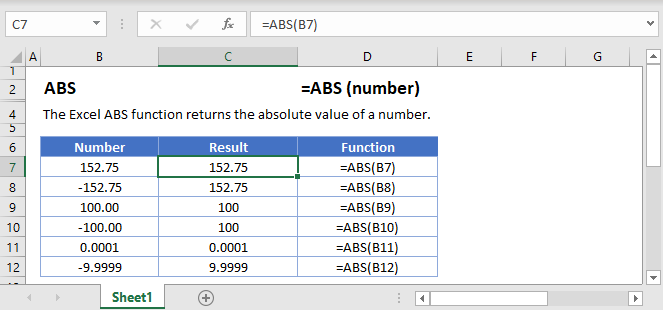ABS Function Overview
The ABS Function Calculates the absolute value of a number. The absolute value is the number’s distance from zero. Example: The absolute value of -9 is 9.

To use the ABS Excel Worksheet Function, select a cell and type: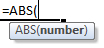(Notice how the formula inputs appear)

### ABS Function Syntax and Inputs:

number – A number.

### Creating a absolute value graph

To create a cosine curve in Excel, we need to first choose our start and end points and then list out a lot of numbers. Let’s go from -1 up to 1 in increments of 0.1.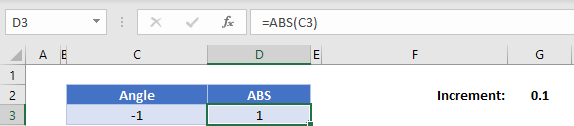Next, we’re going to add the 0.1 onto the angle and then calculate the cosine of that angle. Use the formula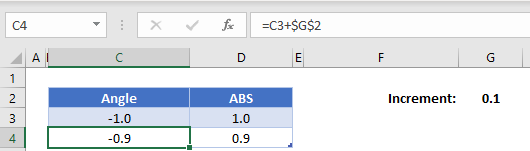(the \$ signs lock G2 so the formula will always reference that 0.1 even if we copy the formula!)

Now highlight both the new angle and cosine function that we’ve calculated, hold the handle, and drag it down until our angle reaches 1.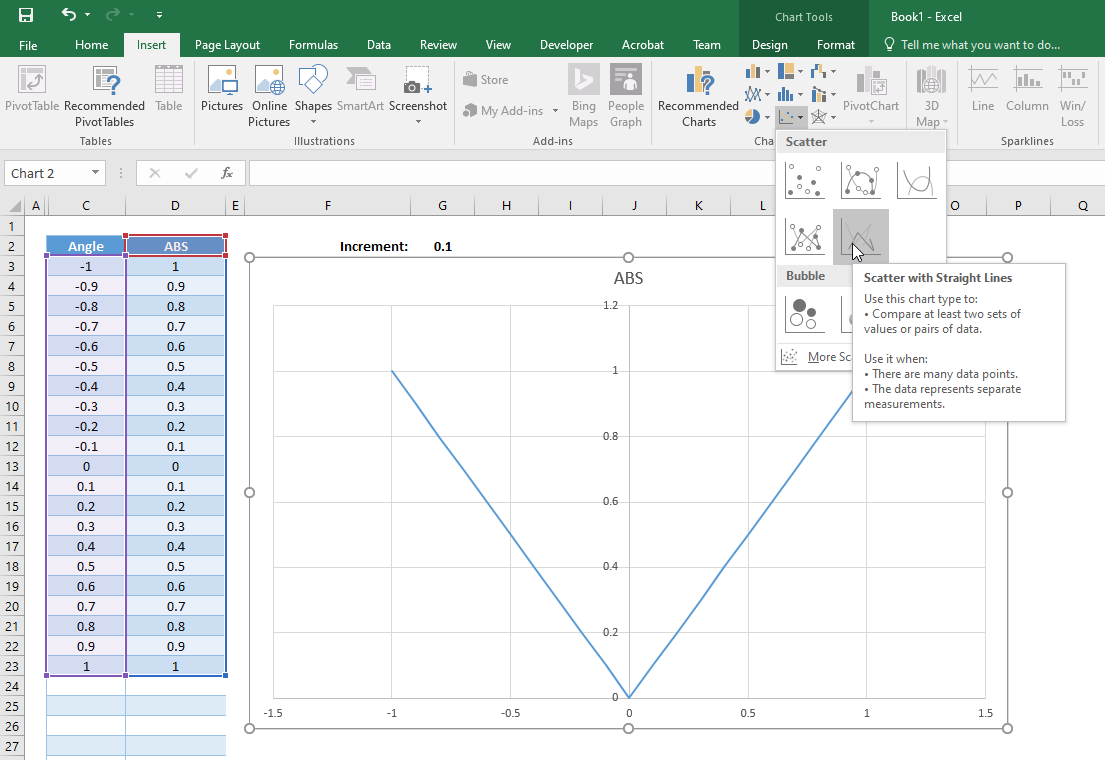If you chose “Scatter with Smooth Lines”, you might notice the graph is a bit wobbly close to (0,0), and it does not create a sharp point as expected at x=0. This is because of our chosen increment of 0.1. To fix this, either use a smaller increment like 0.01 or choose “Scatter with Straight Lines”.

The ABS Function works exactly the same in Google Sheets as in Excel:

Highlight the entire range of both angles and cosines, click insert, find the graphs and select “Scatter with Straight Lines”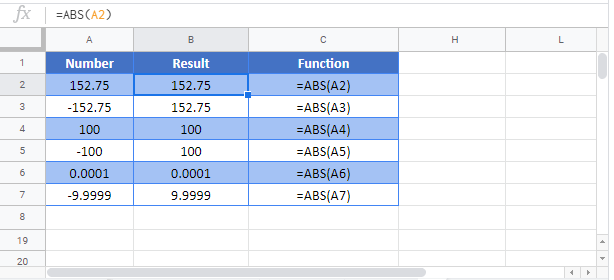## ABS Examples in VBA

You can also use the ABS function in VBA. Type:` application.worksheetfunction.abs(number)`

Running the following VBA statements

will create the following output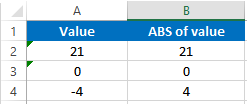For the function arguments (number, etc.), you can either enter them directly into the function, or define variables to use instead.